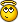## Recommended Posts

general maths:

```Func GetRoot(\$num, \$root)
If IsNumber(\$num) = 0 Or IsNumber(\$root) = 0 Or \$num < 0 Then
SetError(1)
Else
Return (\$num ^ (1 / \$root))
EndIf
EndFunc

Func CalculateCrossSum(\$Num)
If IsNumber(\$Num) = 0 Or \$Num < 0 Then
SetError(1)
Else

Local \$StartNum = 0
Local \$NumString = String(\$Num)
Local \$Split = StringSplit(\$NumString, "")

For \$i = 1 To \$Split
\$StartNum = \$StartNum + Number(\$Split[\$i])
Next

Return \$StartNum
EndIf
EndFunc```

i dont know if CrossSum is the right wordbut i think you should know what it should meanand my Prime Number functions:

```Func CountPrimeFactors(\$num)
If IsNumber(\$num) = 0 Or IsInt(\$num) = 0 Then
SetError(1)
ElseIf \$num < 0 Then
\$num = \$num * -1
Else

Local \$i = 2
Local \$count = 1

While \$i <= \$num / 2
If IsInt(\$num / \$i) Then
\$num = \$num / \$i
\$count = \$count + 1
\$i = 2
Else
\$i = \$i + 1
EndIf
WEnd

Return \$count
EndIf
EndFunc

If IsNumber(\$num) = 0 Or (\$num < 2 And \$num > 0) Or IsInt(\$num) = 0 Then
SetError(1)
ElseIf \$num < 0 Then
\$num = \$num * -1
Else

Local \$i = 2
Local \$bool = 1

If \$num <> 2 Then
While \$i <= \$num / 2 And \$bool = 1
If IsInt(\$num / \$i) Then
\$bool = 0
Else
\$i = \$i + 1
EndIf
WEnd
EndIf

Return \$bool
EndIf
EndFunc

Func GetPrimeFactors(\$num)
If IsNumber(\$num) = 0 Or \$num <= 0 Or IsInt(\$num) = 0 Then
SetError(1)
ElseIf \$num < 0 Then
\$num = \$num * -1
Else

Local \$PrimeFactors[CountPrimeFactors(\$num) + 1]
Local \$i = 1
Local \$j = 2

\$PrimeFactors = UBound(\$PrimeFactors) - 1

While \$i <= \$PrimeFactors
If IsInt(\$num / \$j) Then
\$PrimeFactors[\$i] = \$j
\$num = \$num / \$j
\$j = 2
\$i = \$i + 1
Else
\$j = \$j + 1
EndIf
WEnd

Return \$PrimeFactors
EndIf
EndFunc```

the last one is the most interesting so heres an example:

```\$num = 123
\$factors = GetPrimeFactors(\$num)
MsgBox(0, "Prime Factors of " & \$num, \$factors)
For \$i = 1 To \$factors
MsgBox(0, \$i & ". Prime Factor of " & \$num, \$factors[\$i])
Next```

GetPrimeFactors returns an array. array contains the number of Prime Factors found. the others the factors. so the first msgbox tells you how many factors where found and the others the value.

hope you like it.

Mike

## Create an account

Register a new account

• ### Recently Browsing   0 members

×

• Wiki

• Back

• #### Beta

• Git
• FAQ
• Our Picks
×
• Create New...